coint
Perform either (1) Johansen’s system cointegration test, (2) Engle-Granger or Phillips-Ouliaris single equation cointegration testing, or (3) Pedroni, Kao, or Fisher panel cointegration testing for the series in the group.
There are three forms for the coint command depending on which form of the test you wish to perform.
Johansen Cointegration Test Syntax
group_name.coint(options) [lag_spec] [@ x1 x2 x3 ...] [@exogsr sx1 sx2 sx3 ...] [@exoglr lx1 lx2 lx3 ...] [@exogboth bx1 bx2 bx3 ...]
uses the coint keyword followed by options, and optionally,
a lag_spec consisting of one or more pairs of lag intervals, where the lag orders are for the differences in the error correction representation of the VEC, not the levels representation of the VAR.
an “@”-sign or “@exogsr” followed by a list of exogenous variables in the short-run equation only
“@exoglr” followed by a list of exogenous variables in the long-run relation only
“@exogboth” followed by a list of exogenous variables in both the long-run relation and the short-run equations
(This type of cointegration testing may be used in a non-panel workfile except when performing Fisher combined testing using the Johansen framework.)
Note that the output for Johansen cointegration tests displays p-values for the rank test statistics. These p-values are computed using the response surface coefficients as estimated in MacKinnon, Haug, and Michelis (1999). The 0.05 critical values are also based on the response surface coefficients from MacKinnon-Haug-Michelis. Note: the reported critical values assume no exogenous variables other than an intercept and trend.
Options for the Johansen Test
Deterministic Trend Option
There are 8 different deterministic trend assumptions that you may specify using the “determ=arg” option.
These cases correspond to whether the intercept (“c”) and the trend (“t”) are either
not included (“n”)
in the long-run cointegrating relation only (“l”)
in the short-run equation only (“s”)
in both the long and short-run equations (“b”)
The values of arg are text shortcuts formed by joining a text shortcut for the intercept specification with a text shortcut for the trend specification.
The individual intercept and trend specifications are formed by joining the “c” and the “t” with the appropriate letter describing inclusion in the long and short-run equations.
For example,
“cb” indicates that the constant is in both the long and short-run equation
“tl” indicates that the trend is in the long-run cointegrating equation only
so that
“cbtl” indicates that the constant is in both the long and short-run and the trend is in the long-run only
Using this convention (along with a special “none” option), we may easily describe options arguments for all 8 deterministic cases:

 cntn, none Case 1: No deterministic terms. Corresponding VAR model has no deterministic terms. cltn Case 2: Restricted constant.Constant only in the cointegrating relations.Corresponding VAR has a constant. cbtn (default) Case 3 (JHJ): Unrestricted constantConstant included both in the short-run equation and (artificially) in the cointegrating relations via orthogonalization. Corresponding VAR has a constant and trend. cstn Case 3: Unrestricted constantConstant only in the short-run equation.Corresponding VAR has a trend. cbtl Case 4 (JHJ): Unrestricted constant and restricted trendConstant included both in the short-run equation and (artificially) in the cointegrating relations via orthogonalization, and trend included only in the cointegrating relations.Corresponding VAR has a constant and trend. cstl Case 4: Unrestricted constant and restricted trendConstant only in the short-run equation, and trend only in the cointegrating relation.Corresponding VAR has a trend. cbtb Case 5 (JHJ): Unrestricted constant and trendConstant and trend both included in the short-run equation and (artificially) in the cointegrating relations via orthogonalization.Corresponding VAR has a constant, linear, and quadratic trend. csts Case 5: Unrestricted constant and trendConstant and trend both included in the short-run equation.Corresponding VAR has a linear and quadratic trend.
or you may use the “determsummary” option to compute tests under all deterministic assumptions.
Other Johansen Options

 determsummary Summarize all deterministic trend cases. restrict Impose restrictions as specified by the “restspec=” option. restspec="spec" Define the restricted VEC specification where spec is a space a space delimited list of VEC coefficient restrictions. m = integer, maxit = integer Maximum number of iterations for restricted estimation (only valid if you choose the restrict option). c = scalar, cvg = scalar Convergence criterion for restricted estimation. (only valid if you choose the restrict option). save = mat_name Stores test statistics as a named matrix object. The save= option stores a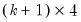matrix, where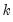is the number of endogenous variables in the VAR. The first column contains the eigenvalues, the second column contains the maximum eigenvalue statistics, the third column contains the trace statistics, and the fourth column contains the log likelihood values. The i-th row of columns 2 and 3 are the test statistics for rank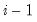. The last row is filled with NAs, except the last column which contains the log likelihood value of the unrestricted (full rank) model. cvtype=ol Display 0.05 and 0.01 critical values from Osterwald-Lenum (1992). This option reproduces the output from version 4. The default is to display critical values based on the response surface coefficients from MacKinnon-Haug-Michelis (1999). Note that the argument on the right side of the equals sign are letters, not numbers 0-1). cvsize=arg (default=0.05) Specify the size of MacKinnon-Haug-Michelis (1999) critical values to be displayed. The size must be between 0.0001 and 0.9999; values outside this range will be reset to the default value of 0.05. This option is ignored if you set “cvtype=ol”. prompt Force the dialog to appear from within a program. p Print results.
Single Equation Test Syntax
group_name.coint(method=arg, options) [@determ determ_spec] [@regdeterm regdeterm_spec]
where

 method=arg Test method: Engle-Granger residual test (“eg”), Phillips-Ouliaris residual test (“po”).
Cointegrating equation specifications that include a constant, linear, or quadratic trends, should use the “trend=” option to specify those terms. If any of those terms are in the stochastic regressors equations but not in the cointegrating equation, they should be specified using the “regtrend=” option.
Deterministic trend regressors that are not covered by the list above may be specified using the keywords @determ and @regdeterm. To specify deterministic trend regressors that enter into the regressor and cointegrating equations, you should add the keyword @determ followed by the list of trend regressors. To specify deterministic trends that enter in the regressor equations but not the cointegrating equation, you should include the keyword @regdeterm followed by the list of trend regressors.
Note that the p-values for the test statistics are based on simulations, and do not account for any user-specified deterministic regressors.
This type of cointegration testing may be used in a non-panel workfile. The remaining options for the single equation cointegration tests are outlined below.
Options for Single Equation Tests
Options for the Engle-Granger Test
The following options determine the specification of the Engle-Granger test (Augmented Dickey-Fuller) equation and the calculation of the variances used in the test statistic.

 trend=arg (default=“const”) Specification for the powers of trend to include in the cointegrating equation: None (“none”), Constant (“const”), Linear trend (“linear”), Quadratic trend (“quadratic”). Note that the specification implies all trends up to the specified order so that choosing a quadratic trend instructs EViews to include a constant and a linear trend term along with the quadratic. regtrend=arg (default=“none”) Additional trends to include in the regressor equations (but not the cointegrating equation): None (“none”), Constant (“const”), Linear trend (“linear”), Quadratic trend (“quadratic”). Only trend orders higher than those specified by “trend=” will be considered.Note that the specification implies all trends up to the specified order so that choosing a quadratic trend instructs EViews to include a constant and a linear trend term along with the quadratic. lag=arg (default=“a”) Method of selecting the lag length (number of first difference terms) to be included in the regression: “a” (automatic information criterion based selection), or integer (user-specified lag length). lagtype=arg (default=“sic”) Information criterion or method to use when computing automatic lag length selection: “aic” (Akaike), “sic” (Schwarz), “hqc” (Hannan-Quinn), “msaic” (Modified Akaike), “msic” (Modified Schwarz), “mhqc” (Modified Hannan-Quinn), “tstat” (t-statistic). maxlag=integer Maximum lag length to consider when performing automatic lag-length selectiondefault=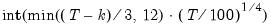whereis the number of coefficients in the cointegrating equation. Applicable when “lag=a”. lagpval=number (default=0.10) Probability threshold to use when performing automatic lag-length selection using a t-test criterion. Applicable when both “lag=a” and “lagtype=tstat”. nodf Do not degree-of-freedom correct estimates of the variances. prompt Force the dialog to appear from within a program. p Print results.
Options for the Phillips-Ouliaris Test
The following options control the computation of the symmetric and one-sided long-run variances in the Phillips-Ouliaris test.
Basic Options

 trend=arg (default=“const”) Specification for the powers of trend to include in the cointegrating equation: None (“none”), Constant (“const”), Linear trend (“linear”), Quadratic trend (“quadratic”). Note that the specification implies all trends up to the specified order so that choosing a quadratic trend instructs EViews to include a constant and a linear trend term along with the quadratic. regtrend=arg (default=“none”) Additional trends to include in the regressor equations (but not the cointegrating equation): None (“none”), Constant (“const”), Linear trend (“linear”), Quadratic trend (“quadratic”). Only trend orders higher than those specified by “trend=” will be considered.Note that the specification implies all trends up to the specified order so that choosing a quadratic trend instructs EViews to include a constant and a linear trend term along with the quadratic. nodf Do not degree-of-freedom correct the coefficient covariance estimate. prompt Force the dialog to appear from within a program. p Print results.
HAC Whitening Options

 lag=arg (default=0) Lag specification: integer (user-specified lag value), “a” (automatic selection). infosel=arg (default=“aic”) Information criterion for automatic selection: “aic” (Akaike), “sic” (Schwarz), “hqc” (Hannan-Quinn) (if “lag=a”). maxlag=integer Maximum lag-length for automatic selection (optional) (if “lag=a”). The default is an observation-based maximum.
HAC Kernel Options

 kern=arg (default=“bart”) Kernel shape: “none” (no kernel), “bart” (Bartlett, default), “bohman” (Bohman), “daniell” (Daniel), “parzen” (Parzen), “parzriesz” (Parzen-Riesz), “parzgeo” (Parzen-Geometric), “parzcauchy” (Parzen-Cauchy), “quadspec” (Quadratic Spectral), “trunc” (Truncated), “thamm” (Tukey-Hamming), “thann” (Tukey-Hanning), “tparz” (Tukey-Parzen). bw=arg (default=“nwfixed”) Bandwidth: “fixednw” (Newey-West fixed), “andrews” (Andrews automatic), “neweywest” (Newey-West automatic), number (User-specified bandwidth). nwlag=integer Newey-West lag-selection parameter for use in nonparametric bandwidth selection (if “bw=neweywest”). bwoffset=integer (default=0) Apply integer offset to bandwidth chosen by automatic selection method (“bw=andrews” or “bw=neweywest”). bwint Use integer portion of bandwidth chosen by automatic selection method (“bw=andrews” or “bw=neweywest”).
Panel Test Syntax
group_name.coint(option)
The coint command tests for cointegration among the series in the group. This form of the command should be used with panel structured workfiles.
Options for the Panel Tests
For panel cointegration tests, you may specify the type using one of the following keywords:

 Pedroni (default) Pedroni (1994 and 2004). Kao Kao (1999) Fisher Fisher - pooled Johansen
Depending on the type selected above, the following may be used to indicate deterministic trends:

 const (default) Include a constant in the test equation.Applicable to Pedroni and Kao tests. trend Include a constant and a linear time trend in the test equation.Applicable to Pedroni tests. none Do not include a constant or time trend.Applicable to Pedroni tests. determ=arg Indicate deterministic trends as detailed above in “Options for the Johansen Test”.Applicable to Fisher tests.

 hac=arg (default=“bt”) Method of estimating the frequency zero spectrum: “bt” (Bartlett kernel), “pr” (Parzen kernel), “qs” (Quadratic Spectral kernel).Applicable to Pedroni and Kao tests. bw=arg (default=“nw”) Method of selecting the bandwidth, where arg may be “nw” (Newey-West automatic variable bandwidth selection), or a number indicating a user-specified common bandwidth.Applicable to Pedroni and Kao tests. lag=arg For Pedroni and Kao tests, the method of selecting lag length (number of first difference terms) to be included in the residual regression. For Fisher tests, a pair of numbers indicating lag. infosel=arg (default=“sic”) Information criterion to use when computing automatic lag length selection: “aic” (Akaike), “sic” (Schwarz), “hqc” (Hannan-Quinn).Applicable to Pedroni and Kao tests. maxlag=int Maximum lag length to consider when performing automatic lag length selection, where int is an integer. The default is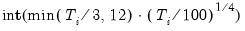where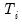is the length of the cross-section.Applicable to Pedroni and Kao tests. disp=arg (default=500) Maximum number of individual results to be displayed. prompt Force the dialog to appear from within a program. p Print results.
Examples
Johansen Test
gr1.coint(determsummary) 1 4
summarizes the results of the Johansen cointegration test for the series in the group GR1 for all specifications of trend. The test equation uses lags of up to order four.
Engle-Granger Test
gr1.coint(method=eg)
performs the default Engle-Granger test on the residuals from a cointegrating equation which includes a constant. The number of lags is determined using the SIC criterion and an observation-based maximum number of lags.
gr1.coint(method=eg, trend=linear, lag=a, lagtype=tstat, lagpval=.15, maxlag=10)
employs a cointegrating equation that includes a constant and linear trend, and uses a sequential t-test starting at lag 10 with threshold probability 0.15 to determine the number of lags.
gr1.coint(method=eg, lag=5)
conducts an Engle-Granger cointegration test on the residuals from a cointegrating equation with a constant, using a fixed lag of 5.
Phillips-Ouliaris Test
gr1.coint(method=po)
performs the default Phillips-Ouliaris test on the residuals from a cointegrating equation with a constant, using a Bartlett kernel and Newey-West fixed bandwidth.